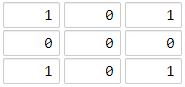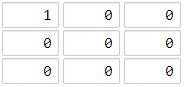##### Welcome to Subscribe On Youtube

Formatted question description: https://leetcode.ca/all/1162.html

# 1162. As Far from Land as Possible (Medium)

Given an N x N grid containing only values 0 and 1, where 0 represents water and 1 represents land, find a water cell such that its distance to the nearest land cell is maximized and return the distance.

The distance used in this problem is the Manhattan distance: the distance between two cells (x0, y0) and (x1, y1) is |x0 - x1| + |y0 - y1|.

If no land or water exists in the grid, return -1.

Example 1:Input: [[1,0,1],[0,0,0],[1,0,1]]
Output: 2
Explanation:
The cell (1, 1) is as far as possible from all the land with distance 2.


Example 2:Input: [[1,0,0],[0,0,0],[0,0,0]]
Output: 4
Explanation:
The cell (2, 2) is as far as possible from all the land with distance 4.


Note:

1. 1 <= grid.length == grid.length <= 100
2. grid[i][j] is 0 or 1

Companies: Uipath

Similar Questions:

## Solution 1. Brute Force

For each water cell, compute its minimal distance to all lands. The maximum of those minimal distances is the answer.

Note that if dist is already smaller than or equal to the current best answer ans, this cell should be skipped because it can’t yield better result (if (dist <= ans) break;).

// OJ: https://leetcode.com/problems/as-far-from-land-as-possible/
// Time: O(MNL) where grid is of size M*N and L is the count of lands.
// Space: O(L)
class Solution {
public:
int maxDistance(vector<vector<int>>& grid) {
vector<pair<int, int>> lands;
int M = grid.size(), N = grid.size(), ans = -1;
for (int i = 0; i < M; ++i) {
for (int j = 0; j < N; ++j) {
if (grid[i][j] == 1) lands.emplace_back(i, j);
}
}
if (lands.empty() || M * N == lands.size()) return -1;
for (int i = 0; i < M; ++i) {
for (int j = 0; j < N; ++j) {
if (grid[i][j] == 1) continue;
int dist = INT_MAX;
for (auto &p : lands) {
int d = abs(p.first - i) + abs(p.second - j);
dist = min(dist, d);
if (dist <= ans) break;
}
ans = max(ans, dist);
}
}
return ans;
}
};


## Solution 2. BFS

Starts from lands, do BFS layer by layer. The maximum layer number you get is the answer.

// OJ: https://leetcode.com/problems/as-far-from-land-as-possible/
// Time: O(MN)
// Space: O(MN)
class Solution {
public:
int maxDistance(vector<vector<int>>& grid) {
queue<pair<int, int>> q;
int M = grid.size(), N = grid.size(), ans = -1;
int dirs = { {0,1},{1,0},{0,-1},{-1,0} };
for (int i = 0; i < M; ++i) {
for (int j = 0; j < N; ++j) {
if (grid[i][j] == 1) q.emplace(i, j);
}
}
if (q.empty() || M * N == q.size()) return -1;
while (q.size()) {
int cnt = q.size();
while (cnt--) {
auto p = q.front();
q.pop();
for (auto &dir : dirs) {
int x = p.first + dir, y = p.second + dir;
if (x < 0 || x >= M || y < 0 || y >= N || grid[x][y]) continue;
q.emplace(x, y);
grid[x][y] = 1;
}
}
++ans;
}
return ans;
}
};


Java

• class Solution {
public int maxDistance(int[][] grid) {
final int WHITE = 0;
final int GRAY = 1;
final int BLACK = 2;
int sideLength = grid.length;
int[][] colors = new int[sideLength][sideLength];
int[][] distances = new int[sideLength][sideLength];
for (int i = 0; i < sideLength; i++) {
for (int j = 0; j < sideLength; j++) {
if (grid[i][j] == 1) {
colors[i][j] = GRAY;
distances[i][j] = 0;
queue.offer(new int[]{i, j});
} else {
colors[i][j] = WHITE;
distances[i][j] = Integer.MAX_VALUE;
}
}
}
if (queue.isEmpty() || queue.size() == sideLength * sideLength)
return -1;
int maxDistance = 0;
int[][] directions = { {-1, 0}, {1, 0}, {0, -1}, {0, 1} };
while (!queue.isEmpty()) {
int[] cell = queue.poll();
int row = cell, column = cell;
int distance = distances[row][column];
maxDistance = Math.max(maxDistance, distance);
for (int[] direction : directions) {
int newRow = row + direction, newColumn = column + direction;
if (newRow >= 0 && newRow < sideLength && newColumn >= 0 && newColumn < sideLength && colors[newRow][newColumn] == WHITE) {
colors[newRow][newColumn] = GRAY;
distances[newRow][newColumn] = Math.min(distances[newRow][newColumn], distance + 1);
queue.offer(new int[]{newRow, newColumn});
}
}
colors[row][column] = BLACK;
}
return maxDistance;
}
}

• // OJ: https://leetcode.com/problems/as-far-from-land-as-possible/
// Time: O(MNL) where grid is of size M*N and L is the count of lands.
// Space: O(L)
class Solution {
public:
int maxDistance(vector<vector<int>>& grid) {
vector<pair<int, int>> lands;
int M = grid.size(), N = grid.size(), ans = -1;
for (int i = 0; i < M; ++i) {
for (int j = 0; j < N; ++j) {
if (grid[i][j] == 1) lands.emplace_back(i, j);
}
}
if (lands.empty() || M * N == lands.size()) return -1;
for (int i = 0; i < M; ++i) {
for (int j = 0; j < N; ++j) {
if (grid[i][j] == 1) continue;
int dist = INT_MAX;
for (auto &p : lands) {
int d = abs(p.first - i) + abs(p.second - j);
dist = min(dist, d);
if (dist <= ans) break;
}
ans = max(ans, dist);
}
}
return ans;
}
};

• class Solution:
def maxDistance(self, grid: List[List[int]]) -> int:
n = len(grid)
q = deque([(i, j) for i in range(n) for j in range(n) if grid[i][j] == 1])
ans = -1
valid = False
while q:
ans += 1
for _ in range(len(q)):
i, j = q.popleft()
for a, b in [[0, 1], [0, -1], [1, 0], [-1, 0]]:
x, y = i + a, b + j
if 0 <= x < n and 0 <= y < n and grid[x][y] == 0:
valid = True
grid[x][y] = 1
q.append((x, y))
return ans if valid else -1

############

# 1162. As Far from Land as Possible
# https://leetcode.com/problems/as-far-from-land-as-possible/

class Solution:
def maxDistance(self, grid: List[List[int]]) -> int:
rows, cols = len(grid), len(grid)
q = collections.deque()

for i in range(rows):
for j in range(cols):
if grid[i][j] == 1:
for dx, dy in ((i + 1, j), (i - 1, j), (i, j + 1), (i, j - 1)):
q.append((dx, dy))

steps = 0
while q:
steps += 1
n = len(q)

for _ in range(n):
i, j = q.popleft()
if 0 <= i < rows and 0 <= j < cols and grid[i][j] == 0:
grid[i][j] = steps

for dx, dy in ((i + 1, j), (i - 1, j), (i, j + 1), (i, j - 1)):
q.append((dx, dy))

return steps - 1 if steps != 1 else -1